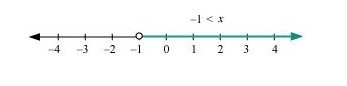# Solve the given inequality and show the graph of the solution on number line: 3(1 – x) < 2 (x + 4)

Question:

Solve the given inequality and show the graph of the solution on number line: $3(1-x)<2(x+4)$

Solution:

$3(1-x)<2(x+4)$

$\Rightarrow 3-3 x<2 x+8$

$\Rightarrow 3-8<2 x+3 x$

$\Rightarrow-5<5 x$

$\Rightarrow \frac{-5}{5}<\frac{5 x}{5}$

\$\Rightarrow-1

The graphical representation of the solutions of the given inequality is as follows.